Search
Document Center

# Automatic parameter tuning with Auto ML

Last Updated: Sep 03, 2018

## Configure parameters

1. Log on to the Machine Learning Platform for AI console.

2. In the left-side navigation pane, click Experiments.

3. Click your target experiment to enter the canvas of the experiment. This topic takes the Weather Prediction experiment as an example.

4. On the upper-left corner of the canvas, select Auto ML > Automatic parameter tuning.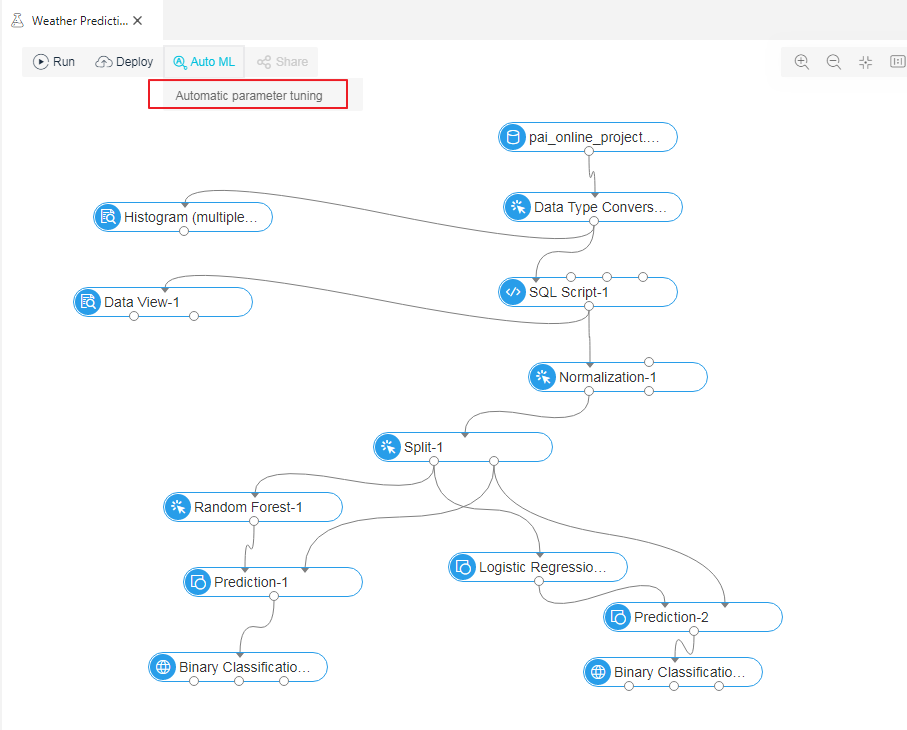5. Select one algorithm for parameter tuning, and then click Next.

Note: You can only select one algorithm to tune at a time.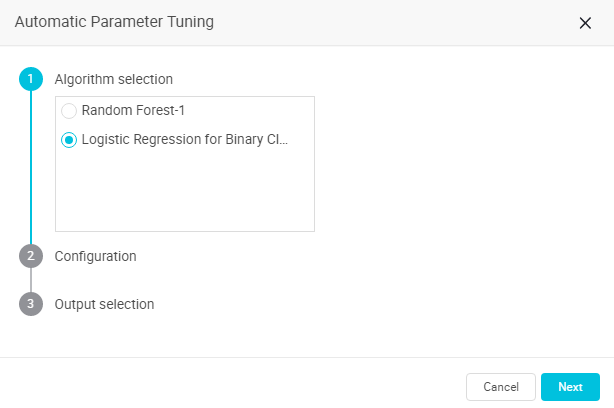6. In the Configuration module, select a parameter tuning method, and then click Next.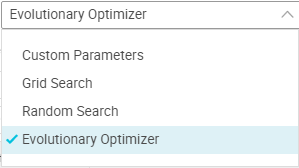Alibaba Cloud Machine Learning Platform for AI provides the following parameter tuning methods:

• Evolutionary Optimizer

Concept:

1. Randomly selects A parameter candidate sets (where A indicates the Number of exploration samples).

2. Takes the N parameter candidate sets with higher evaluation indicators as the parameter candidate sets of the next iteration.

3. Continues the exploration within R times (where R indicates Convergence coefficient) as the standard deviation range around these parameters to explore new parameter sets. The new parameter sets replace the last A-N parameter sets by the evaluation indicator in the previous round.

4. Iterates the exploration for M rounds (where M indicates Number of searches) until the optimal parameter set is found, according to the preceding logic.

According to the preceding principle, the final number of models is A+(A-N)*M.

Note: Continues the exploration within R times (where R indicates Convergence coefficient) as the standard deviation range around these parameters to explore new parameter sets to replace the last A-N parameter sets by the evaluation indicator in the previous round. The first value of N is A/2-1, which defaults to N/2-1 during the iteration (decimal values are rounded up to an integer).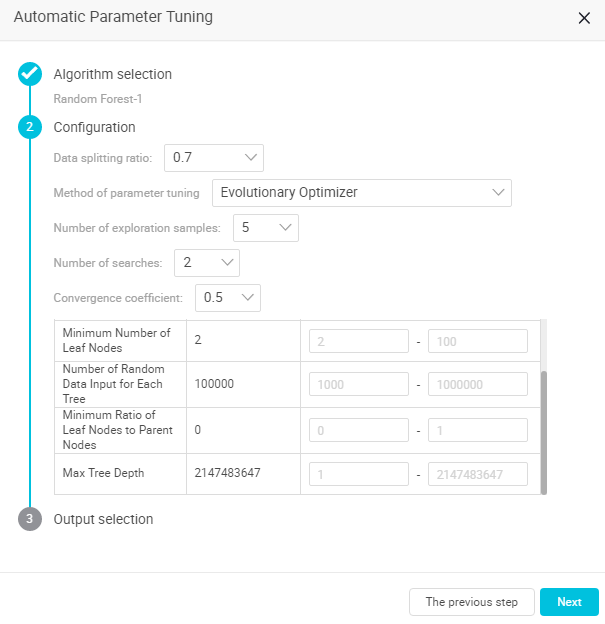• Data splitting ratio: Splits input data sources into training and evaluation sets. 0.7 indicates that 70% of the data is for training a model, and the remaining 30% of data is for evaluation.

• Number of exploration samples: The number of parameter sets of each iteration. The higher the number, the greater the accuracy, the larger the calculation. The value range is 5-30.

• Number of searches: The number of iterations. The higher the number of iterations, the greater the search accuracy, the larger the calculation. The value range is 1-10.

• Convergence coefficient: Tunes the exploration ranges (R times the standard deviation range search). The smaller the range, the faster the convergence (however, optimal parameters may be missed). The value range is 0.1-1 (one floating point after the decimal point).

• You must enter the tuning ranges for each parameter. If the current parameter range is not configured, the parameter range is set by default.
• Random Search

Concept:

1. Each parameter randomly selects a value within its range.

2. Enters random values into a set of parameters for model training.

3. Performs M rounds (where M indicates the number of iterations) and then orders the output models.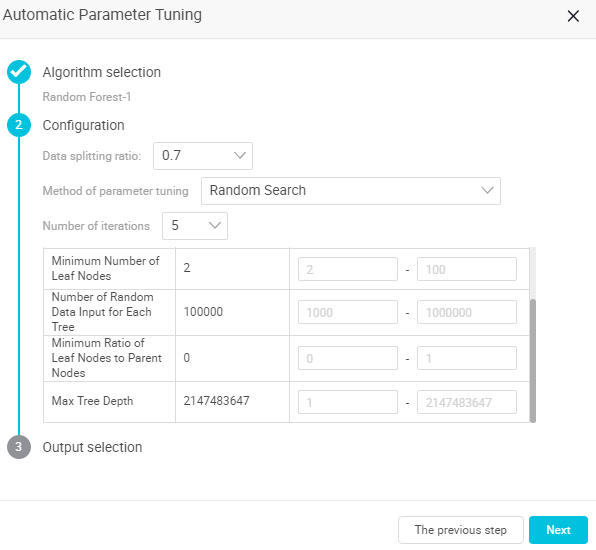• Number of iterations: Indicates the number of searches in the configured interval. The value range is 2 to 50.

• Data splitting ratio: Splits input data sources into training and evaluation sets. 0.7 indicates that 70% of the data is for training a model, and the remaining 30% of data is for evaluation.

• You must enter the tuning ranges for each parameter. If the current parameter range is not configured, the parameter range is set by default.

• Grid Search

Concept:

1. Splits the value range of each parameter into N segments (grid split score).

2. Randomly takes a value from the N segments. Assuming that there are M parameters, N^M parameter groups can be combined.

3. According to the N^M parameter groups, N^M models are generated by training. The models are then ordered.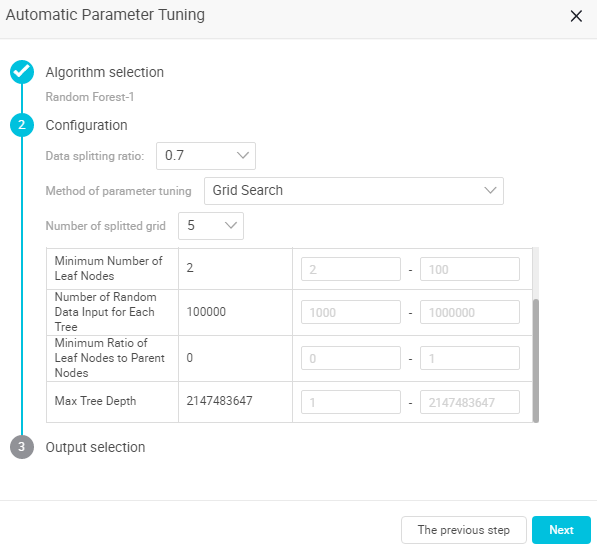• Number of splitted grid: Indicates the number of split grids. The value range is 2-10.

• Data splitting ratio: Splits input data sources into training and evaluation sets. 0.7 indicates that 70% of the data is for training a model, and the remaining 30% of data is for evaluation.

• You must enter the tuning ranges for each parameter. If the current parameter range is not configured, the parameter range is set by default.

• Custom Parameters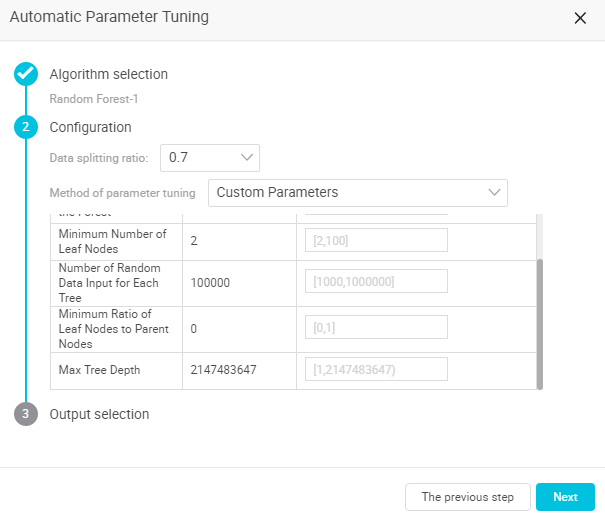• You can enumerate parameter candidate sets. The system then helps you to score all the combinations of the candidate sets.

• You can define enumeration ranges. Parameters are separated by commas. If the ranges are not configured, the parameters are tuned by default.

7. In the Output selection module, configure model Output parameters, and then click Next.

• Evaluation criteria: Select one evaluation standard from the following four dimensions: AUC, F1 Score, Precision, and RECALL.

• Number of models to be saved: You can save up to five models. The system ranks models and save the top ranked models according to the number entered in the Number of models to be saved field.

• Pass down the model: The switch is turned ON by default. If the switch is OFF, the model generated by the default parameters of the current component are passed down to the node of the subsequent component. If the switch is ON, the optimal model generated by automatic parameter tuning are passed down to the node of the subsequent component.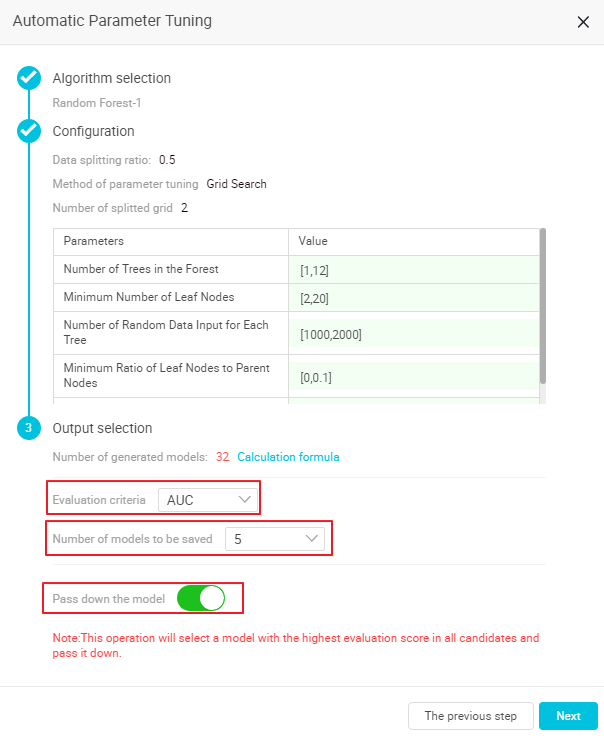8. In the upper-left corner of the canvas, click Run to run the automatic parameter tuning algorithm.

Note: After the preceding configuration is run, the Auto ML switch of the related algorithm is turned ON. You can turn the switch ON or OFF as required.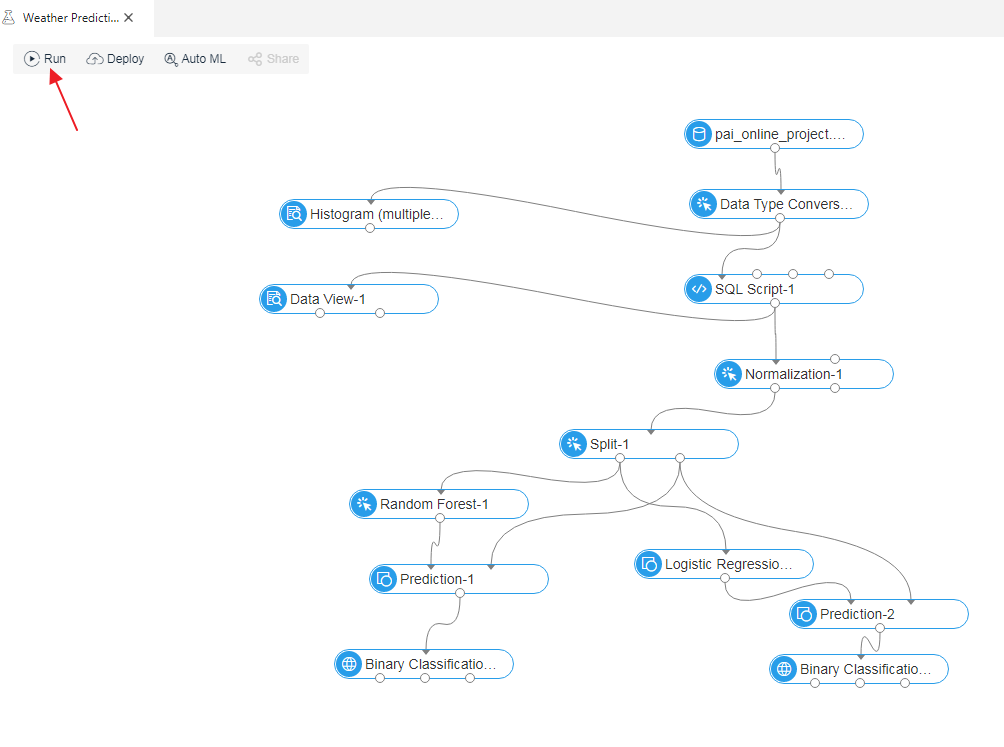9. (Optional) Right-click a model component, and then select Edit the AutoML parameters to modify its Auto ML configuration parameters.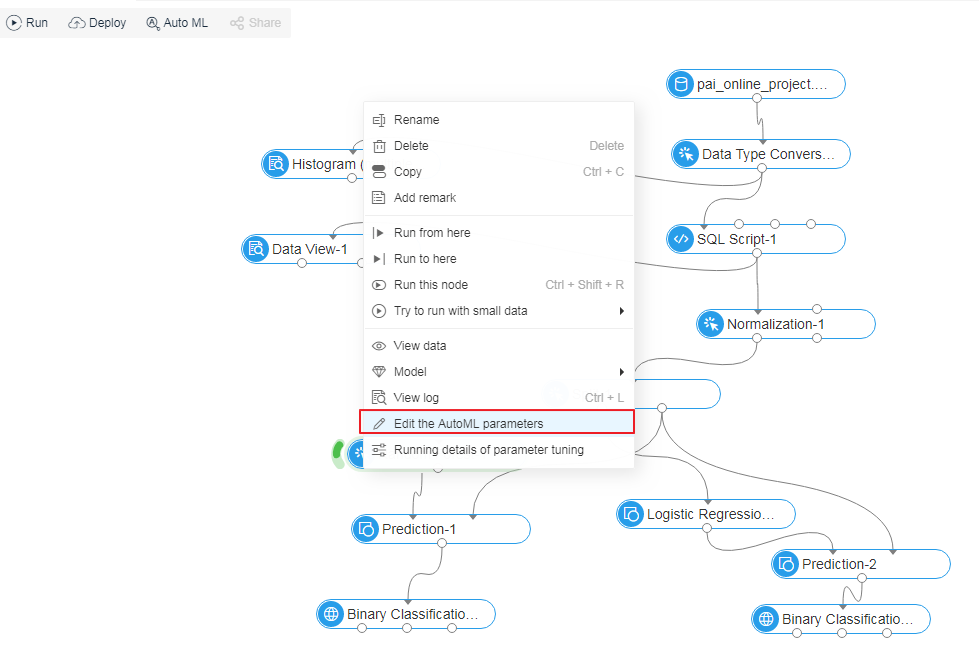## Output model display

1. During parameter tuning, right click the target model component and then select Running details of parameter tuning.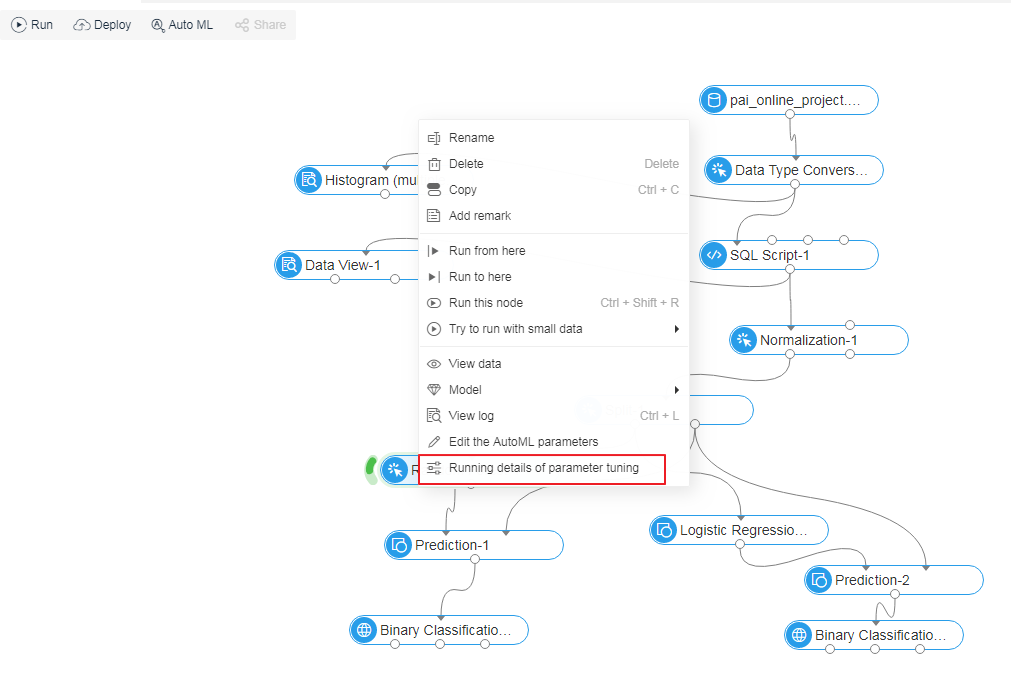2. In the AutoML-Details of Automatic Parameter Tuning pane, click Indicator Data to view the current tuning progress and the running status of each model.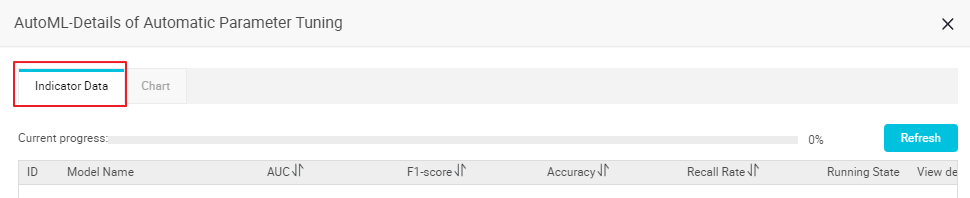3. You can order candidate models according to indicators (AUC, F1-score, Accuracy, and Recall Rate).

4. In the View details column, you can click Log or Parameter to view the logs and parameters of each candidate model.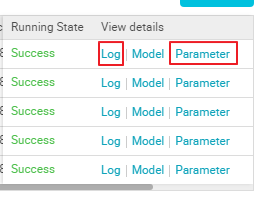## Parameter tuning effect display

Click Chart on the Indicator Data page to view the Model evaluation & comparison and Effect Comparison of Hyper-parameters Iteration charts.

For example, you can view the growth trend of the evaluation indicators of updated parameters in Effect Comparison of Hyper-parameters Iteration as shown in the following figure: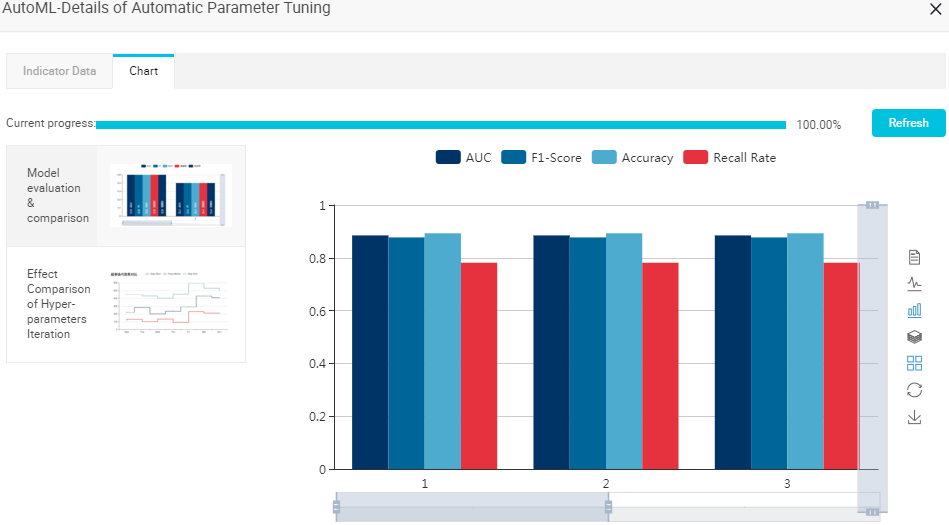## Model storage

1. In the left-side navigation pane, click Models.

2. Click My Experiments.

3. Click the corresponding experiment folder to view the model saved with Auto ML.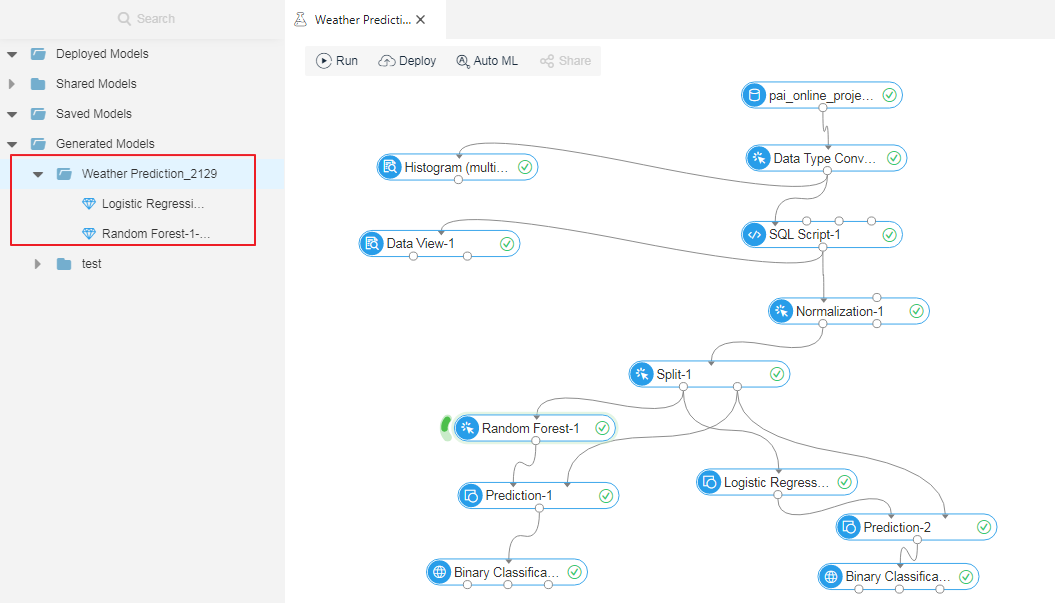4. (Optional) You can apply a model to other experiments by dragging the model to the canvas of the target experiment.